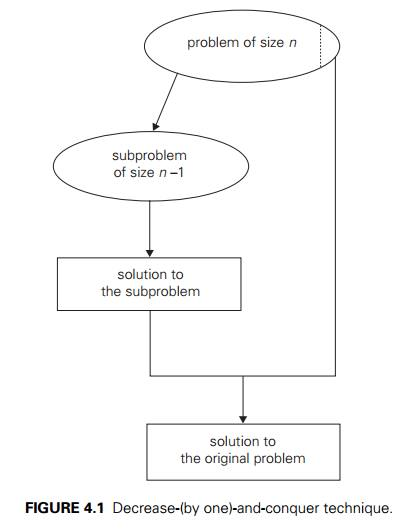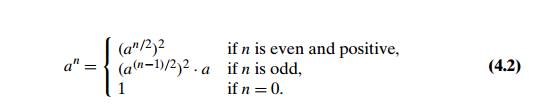Home | | Design and Analysis of Algorithms | Decrease and Conquer

# Decrease and Conquer

The decrease-and-conquer technique is based on exploiting the relationship between a solution to a given instance of a problem and a solution to its smaller instance.

Decrease-and-Conquer

Plutarch says that Sertorius, in order to teach his soldiers that perseverance and wit are better than brute force, had two horses brought before them, and set two men to pull out their tails. One of the men was a burly Hercules, who tugged and tugged, but all to no purpose; the other was a sharp, weasel-faced tailor, who plucked one hair at a time, amidst roars of laughter, and soon left the tail quite bare.

—E. Cobham Brewer, Dictionary of Phrase and Fable, 1898

The decrease-and-conquer technique is based on exploiting the relationship between a solution to a given instance of a problem and a solution to its smaller instance. Once such a relationship is established, it can be exploited either top down or bottom up. The former leads naturally to a recursive implementa-tion, although, as one can see from several examples in this chapter, an ultimate implementation may well be nonrecursive. The bottom-up variation is usually implemented iteratively, starting with a solution to the smallest instance of the

problem; it is called sometimes the incremental approach. There are three major variations of decrease-and-conquer:

decrease by a constant decrease by a constant factor variable size decrease

In the decrease-by-a-constant variation, the size of an instance is reduced by the same constant on each iteration of the algorithm. Typically, this constant is equal to one (Figure 4.1), although other constant size reductions do happen occasionally.

Consider, as an example, the exponentiation problem of computing an where a  = 0 and n is a nonnegative integer. The relationship between a solution to an instance of size n and an instance of size n 1 is obtained by the obvious formula an = an1 . a. So the function f (n) = an can be computed either “top down” by using its recursive definitionor “bottom up” by multiplying 1 by a n times. (Yes, it is the same as the brute-force algorithm, but we have come to it by a different thought process.) More interesting examples of decrease-by-one algorithms appear in Sections 4.1–4.3.

The decrease-by-a-constant-factor technique suggests reducing a problem instance by the same constant factor on each iteration of the algorithm. In most applications, this constant factor is equal to two. (Can you give an example of such an algorithm?) The decrease-by-half idea is illustrated in Figure 4.2.

For an example, let us revisit the exponentiation problem. If the instance of size n is to compute an, the instance of half its size is to compute an/2, with the obvious relationship between the two: an = (an/2)2. But since we consider here instances with integer exponents only, the former does not work for odd n. If n is odd, we have to compute an1 by using the rule for even-valued exponents and then multiply the result by a. To summarize, we have the following formula:If we compute an recursively according to formula (4.2) and measure the algo-rithm’s efficiency by the number of multiplications, we should expect the algorithm to be in  (log n) because, on each iteration, the size is reduced by about a half at the expense of one or two multiplications.

A few other examples of decrease-by-a-constant-factor algorithms are given in Section 4.4 and its exercises. Such algorithms are so efficient, however, that there are few examples of this kind.

Finally, in the variable-size-decrease variety of decrease-and-conquer, the size-reduction pattern varies from one iteration of an algorithm to another. Eu-clid’s algorithm for computing the greatest common divisor provides a good ex-ample of such a situation. Recall that this algorithm is based on the formula

gcd(m, n) = gcd(n, m mod n).

Though the value of the second argument is always smaller on the right-hand side than on the left-hand side, it decreases neither by a constant nor by a constant factor. A few other examples of such algorithms appear in Section 4.5.

Study Material, Lecturing Notes, Assignment, Reference, Wiki description explanation, brief detail
Introduction to the Design and Analysis of Algorithms : Decrease and Conquer : Decrease and Conquer |

Related Topics